# Texas Go Math Grade 6 Module 10 Answer Key Generating Equivalent Numerical Expressions

Refer to our Texas Go Math Grade 6 Answer Key Pdf to score good marks in the exams. Test yourself by practicing the problems from Texas Go Math Grade 6 Module 10 Answer Key Generating Equivalent Numerical Expressions.

## Texas Go Math Grade 6 Module 10 Answer Key Generating Equivalent Numerical Expressions

Find the product.

Question 1.
992 × 16
Given expression = 992 × 16
Rewrite the given expression as a product of a number and a difference, therefore = 16(1000 – 8)
Apply distributive property to expand the parenthesis = 16000 – 128

Evaluate = 15872
9920 × 16 = 15872

Question 2.
578 × 27
Solution to this example is given belowFinal Solution = 15,606

Question 3.
839 × 65
Given expression = 839 × 65
Rewrite the given expression as a product of a number and a difference, therefore = 65(800 + 39)
Apply distributive property to expand the parenthesis = 52000 + 2535

Evaluate = 54535
839 × 65 = 54535

Question 4.
367 × 23
Solution to this example is given below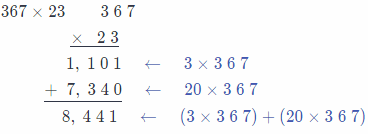Final Solution = 8,441

Find the product.

Question 5.
7 × 7 × 7
Solution to this example is given below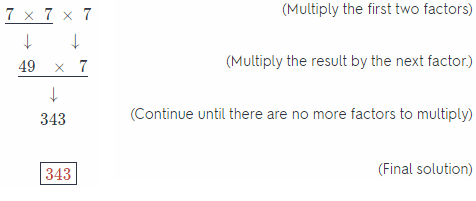Question 6.
3 × 3 × 3 × 3
Solution to this example is given below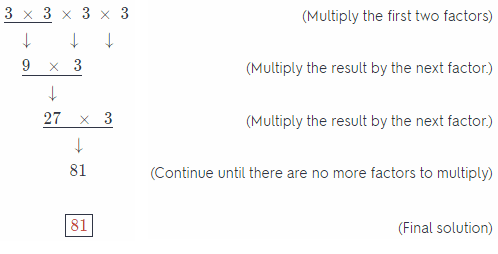Question 7.
6 × 6 × 6 × 6 × 6
Solution to this example is given below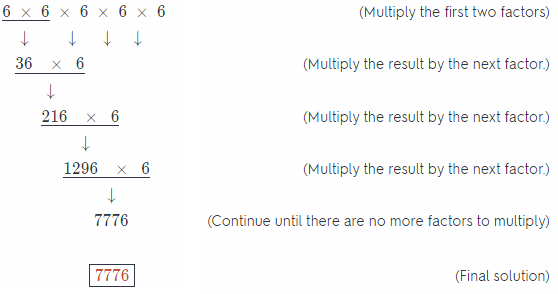Question 8.
2 × 2 × 2 × 2 × 2 × 2 × 2
Solution to this example is given below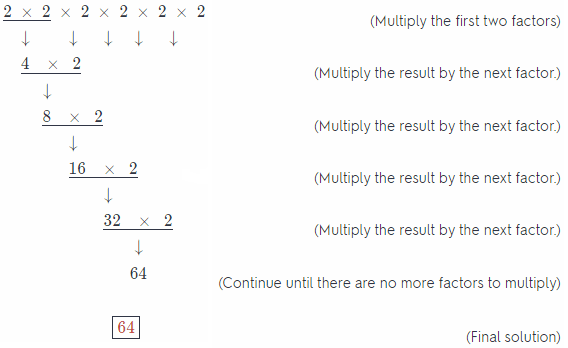Divide.

Question 9.
20 ÷ 4
Solution to this example is given below
20 ÷ 4 = ?
Think: 4 times what number equals 20?
⇒ 4 × 5 = 20
⇒ 20 ÷ 4 = 5
So, 20 ÷ 4 = 5.

Question 10.
21 ÷ 7
Solution to this example is given below
21 ÷ 7 = ?
Think: 7 times what number equals 20?
⇒ 7 × 3 = 21
⇒ 21 ÷ 7 = 3
So, 21 ÷ 7 = 3.

Question 11.
42 ÷ 7
Solution to this example is given below
42 ÷ 7 = ?
Think: 7 times what number equals 20?
⇒ 7 × 6 = 42
⇒ 42 ÷ 7 = 6
So, 42 ÷ 7 = 6.

Question 12.
56 ÷ 8
Solution to this example is given below
56 ÷ 8 = ?
Think: 8 times what number equals 20?
⇒ 8 × 7 = 56
⇒ 56 ÷ 8 = 7
So, 56 ÷ 8 = 7.

Visualize Vocabulary
Use the words to complete the graphic. You may put more than one word in each box.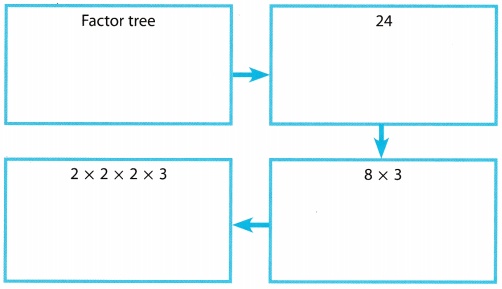Understand Vocabulary

Complete the sentences using the preview words.

Question 1.
A number that is formed by repeated multiplication by the same factor is a _________________ .
Power

Question 2.
A rule for simplifying expressions is _____________________